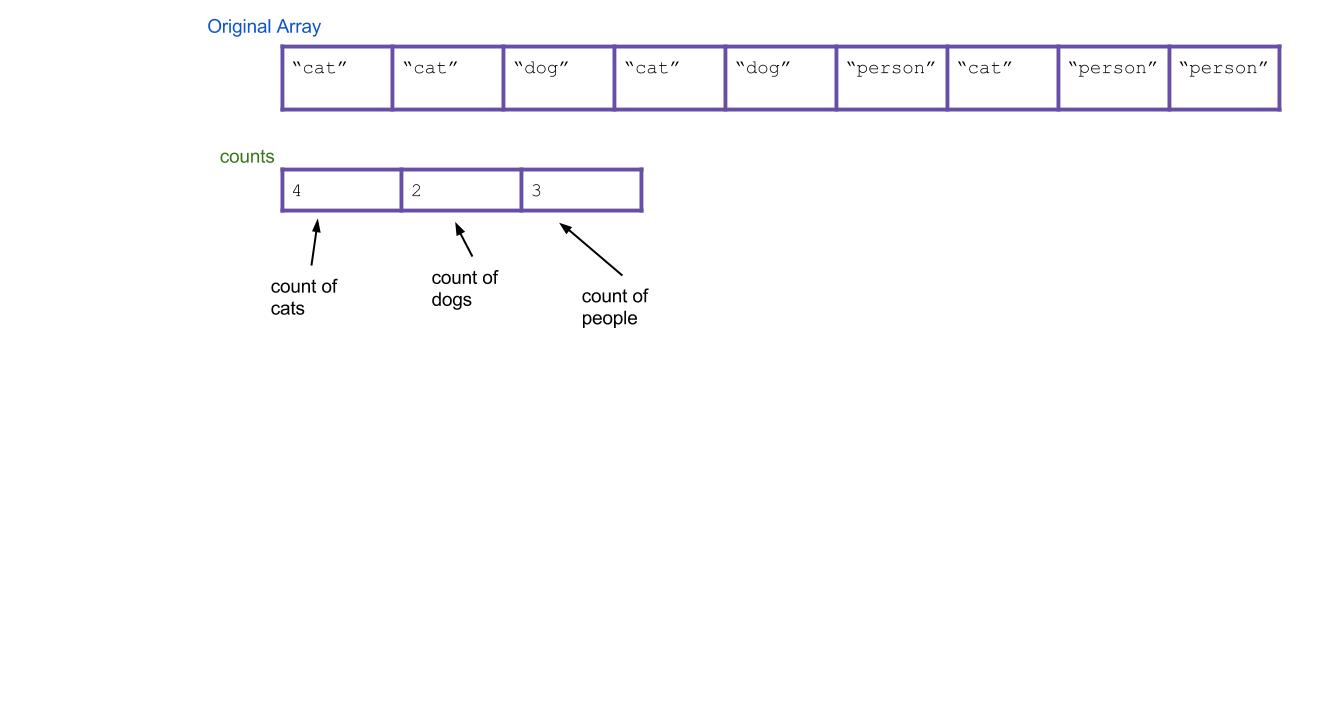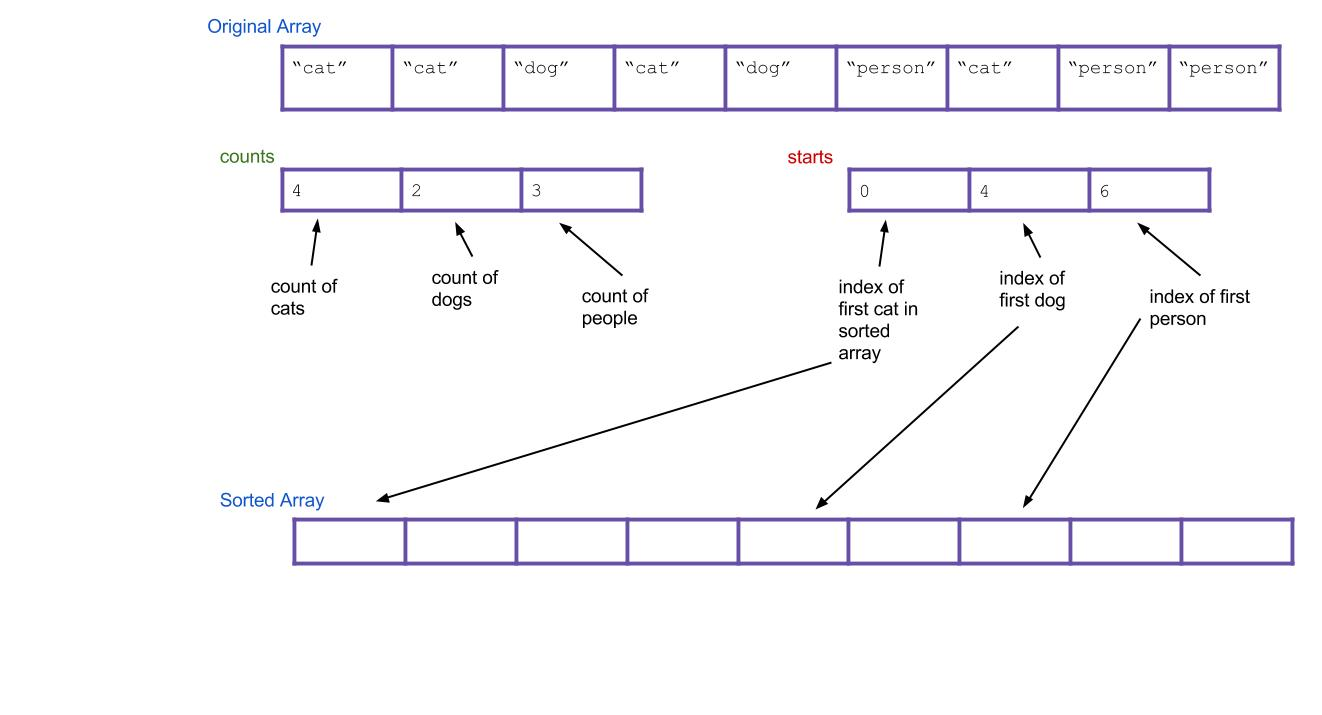Lab 13: Counting Sorts

## Prelude

This week in lab you're going to be writing Counting sort and a form of Radix sort. This lab includes explanatory material drawn from the summer 61BL labs to reinforce the lecture material on counting-based sorts, as well as give background to the problems you're trying to solve. If you're not interested in reading the background or you already feel comfortable with the material, feel free to scroll to the deliverables section at the bottom of the lab to know what you need to turn in.

## Counting Sort

Suppose you have an array of a million Strings, but you happen to know that there are only three different varieties of Strings in it: "Cat", "Dog", and "Person". You want to sort this array to put all the cats first, then all the dogs, then the people. How would you do it? You could use merge sort or Quicksort and get a runtime proportional to N log N, where N is ~one million. Can you do better?

We think you can. Take a step back and don't think too hard about it. What's the simplest thing you could do?

We propose an algorithm called counting sort. For the above example, it works like this:

1. First create an int array of size three. Call it the `counts` array. It will count the total number of each type of String.
2. Iterate through your array of animals. Every time you find a cat, increment `counts` by 1. Every time you find a dog, increment `counts` by 1. Every time you find a person, increment `counts` by 1. As an example, the result could be this:3. Next, create a new array that will be your sorted array. Call it `sorted`.4. Think about it: based on your `counts` array, can you tell where the first dog would go in the new array? The first person? Create a new array, called `starts`, that holds this information. For our example, the result is:5. Now iterate through all of your Strings, and put them into the right spot. When I find the first cat, it goes in `sorted[starts]`. When I find the first dog, it goes in `sorted[starts]`. What about when I find the second dog? It goes in `sorted[starts+1]`, of course. Or, an alternative: I can just increment `starts` every time I put a dog. Then the next dog will always go in `sorted[starts]`.

Here's what everything would look like after completing the algorithm. Notice that the values of `starts` have been incremented along the way.Does the written explanation of counting sort seem complicated? Here is a pretty cool animated version of it that might make it more intuitive.

In this example we arbitrarily decided which index represented "Cat" and "Dog" and "Person", but is there a systematic way to do this? If you are guaranteed to only be sorting positive integers, the answer is yes: count the occurences of `i` in `counts[i]`.

In `CountingSort.java`, we've given you an implementation of this type of positive-integer counting sort. Look at and try running `CountingSortTester` and you'll see that the provided `naiveCountingSort` cannot handle an array with negative numbers.

Fill in the `betterCountingSort` method so that it still does a counting based sort, but also handles negative numbers gracefully.

We've given you some tests in `CountingSortTester`, but if you're interested (this is option), try writing a test that causes your `betterCountingSort` to fail.

The radix of a number system is the number of values a single digit can take on. Binary numbers form a radix-2 system; decimal notation is radix-10. Any radix sort examines elements in passes, one pass for (say) the rightmost digit, one for the next-to-rightmost digit, and so on.

A key realization is the following: given two three-digit numbers (say, 536 and 139), it is possible to sort these numbers one digits-place at a time. There are two ways to do this:

• First sort everything on the least important (right-most) digit. Then sort everything on the next digit to the left. Continue, until you reach the highest digit. This strategy requires the sorts to be stable.
• First sort everything on the high key. Group all the items with the same high key into buckets. Recursively radix sort each bucket on the next highest key. Concatenate your buckets back together.

Here's an example of using the first strategy. Imagine we have the following numbers we wish to sort:

356, 112, 904, 294, 209, 820, 394, 810

First we sort them by the first digit:

820, 810, 112, 904, 294, 394, 356, 209

Then we sort them by the second digit, keeping numbers with the same second digit in their order from the previous step:

904, 209, 810, 112, 820, 356, 294, 394

Finally, we sort by the third digit, keeping numbers with the same third digit in their order from the previous step:

112, 209, 294, 356, 394, 810, 820, 904

All done!

Hopefully it's not hard to see how these can be extended to more than three digits. The first strategy is known as LSD radix sort, and the second strategy is called MSD radix sort. LSD and MSD stand for least significant digit and most significant digit respectively, reflecting the order in which the digits are considered. Here's some pseudocode for the first strategy:

``````public static void LSDRadixSort(int[] arr) {
for (int d = 0; d < numDigitsInAnInteger; d++) {
stableSortOnDigit(arr, d);
}
}``````

(the 0 digit is the smallest digit, or the one furthest to the right in the number)

The pseudocode for the second strategy isn't so clean to write, but its easy to see how it works in practice, let's sort that same list above by MSD RadixSort. Notice how our sort-on-digit doesn't have to be stable this time.

356, 112, 904, 294, 209, 820, 394, 810

First we sort them by the first digit, splitting the list into "buckets" representing numbers with the same value in the digit we sorted on:

, [294, 209], [394, 356], [810, 820], 

For each bucket, recursively do the same thing on the next-less-signifigant digit. For purposes of clarity, let's just look at the 300s bucket. Which is:

394, 356

This now gets sorted and bucketed by the second-most-signifigant-digit:

, 

Finally, we concatenate together and return the concatenated list. MSD is a little easier to conceptualize, but turns out to be a little tougher to implement, especially if you care about efficiency.

In this part of lab you'll write an implementation of radix sort for ASCII Strings. Normally, if we just had decimal numbers, we would say that we would have a radix of 10 (R = 10) since there are 10 possible digits at each index, [0, 1, 2, 3, 4, 5, 6, 7, 8, 9]. It is important to note that the time Radix Sort takes does depend on the length of the longest value it has to sort. We consider running Radix Sort to be linear time for integers in Java because the number of digits allowed for an integer is limited (10 digits max) which means we will at most have to do 10N iterations.

For our purposes in this lab, we are going to be sorting ASCII Strings which have 256 possible characters (numbered 0-255) and are of variable length. In JAVA, you can get the ASCII code for a character by casting the `char` as an `int` (`int i = (int)'a'`) and get the character from the ASCII code by casting the other way (`char a = (char)97`). You may implement either MSD (most significant digit) or LSD (least significant digit). MSD Radix is conceptually simpler, but a litter uglier to code (and slower). LSD Radix is harder to conceptualize, but easier to implement (and fast). Pick which one you'd prefer.

Since we have 256 characters to use, we have a radix of 256 (R = 256). Write the method 'sort' in `RadixSort.java` that will sort the list of ASCII Strings that is passed in and return the sorted list. Make sure the method is NON-destructive (so the original list cannot change). Feel free to add any helper methods you want (you can also use your counting sort implementation). Here is a great tool for seeing how Radix sort works visually.

Keep in mind that Radix Sort on Strings runs in `O(N*M)` time where `N` is the number of Strings and `M` is the length of the longest String. HINT: Remember ASCII codes start from 0, not 1.

Extra for experts (optional): Compare the runtime of your Radix sort compared to `Arrays.sort`. Which is faster for short arrays? Long arrays? Do the values in the array matter?

## Deliverables

Implement `betterCountingSort` in `CountingSort.java` and `sort` in `RadixSort.java`. If you're feeling like that was easy, try implementing alternatve forms of Radix sort!

Note that both sorting algorithms are non destructive!!!